GCSEKS3Level 4-5Level 6-7AQAEdexcelOCR

## Quadratic Graphs and Other Graphs

This topic includes graphs which are not straight  lines.

These include, quadratic graphs, cubic graphsreciprocal graphs and exponential graphs.

You will need to be able to identify and plot these graphs.

You need to be happy with the following topics:

Level 4-5GCSEAQAEdexcelOCR

Quadratic graphs have the general form

$\textcolor{red}{a}x^2 + \textcolor{limegreen}{b}x+\textcolor{blue}{c}$

These form a $\bigcup$ or $\bigcap$ shape, examples are shown below: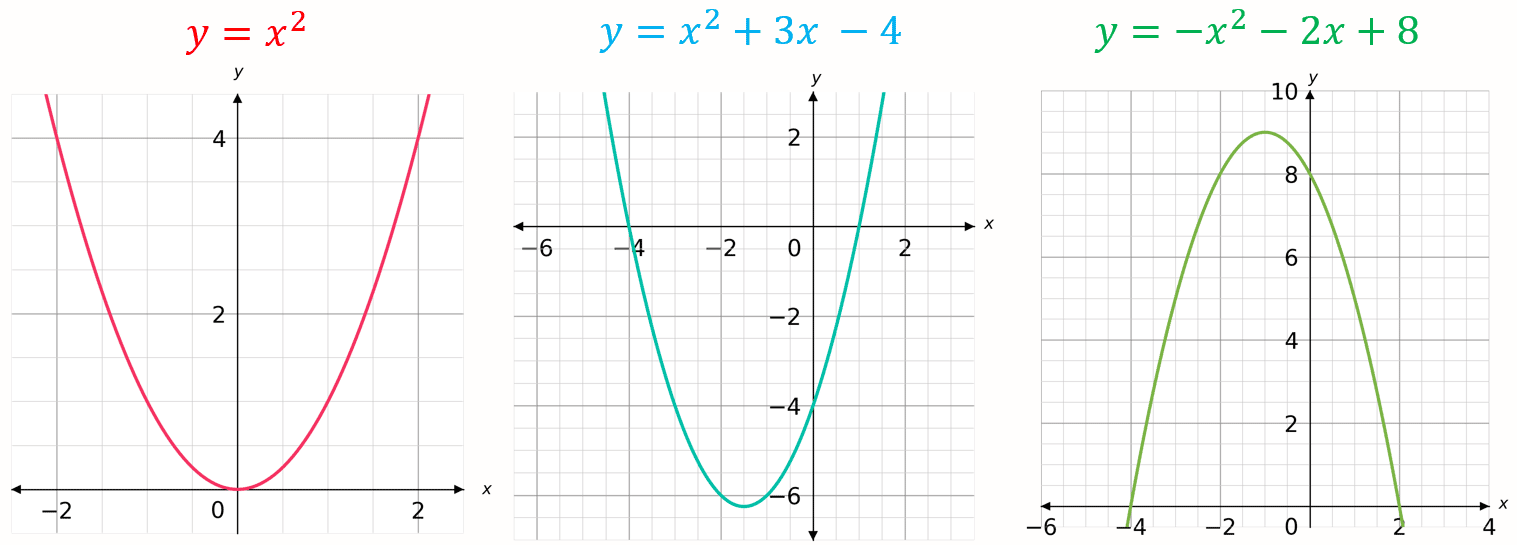Note: $\textcolor{limegreen}{b}$ and $\textcolor{blue}{c}$ can be zero, as is the case with $y=x^2$

Level 4-5GCSEKS3AQAEdexcelOCR

## Cubic Graphs

Cubic graphs have the general form

$\textcolor{red}{a}x^3 + \textcolor{limegreen}{b}x^2+\textcolor{blue}{c}x +\textcolor{maroon}{d}$

These form $S$ shape in the middle.

Note: Sometimes this $S$ can be fairly flat, e.g.

$\textcolor{red}{2}x^3 + \textcolor{limegreen}{3}x^2 + \textcolor{blue}{x}$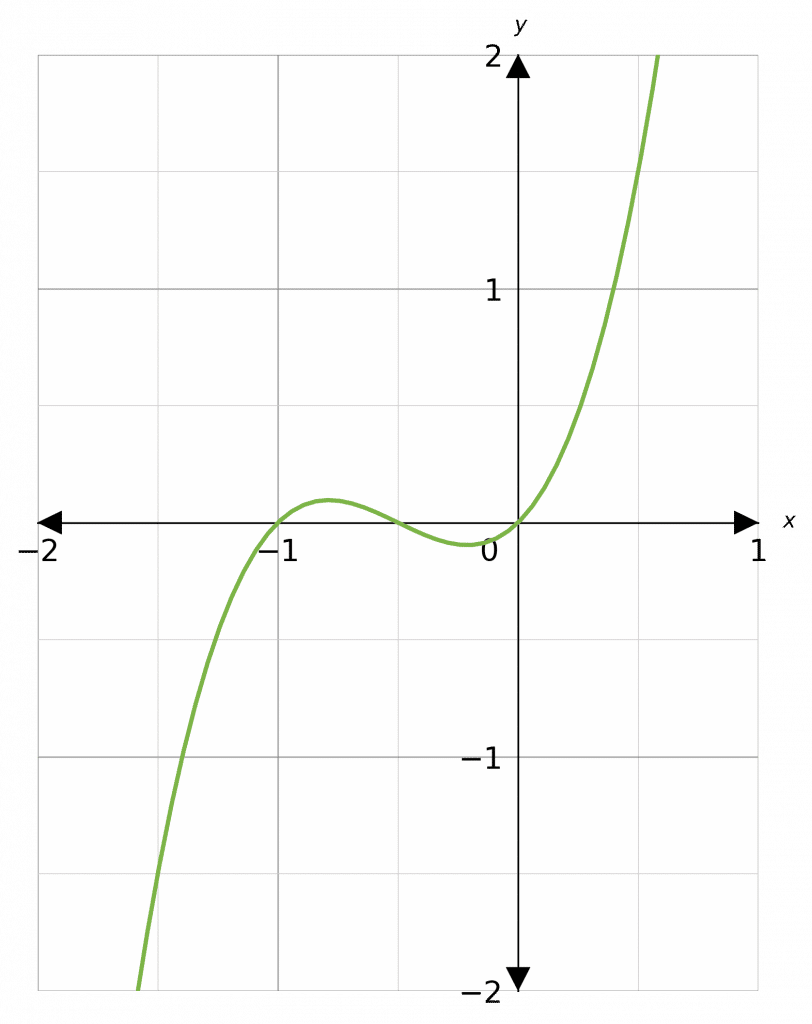Level 4-5GCSEAQAEdexcelOCR
Level 4-5GCSEAQAEdexcelOCR

## Interpreting Roots and Intercepts

The roots of a quadratic are the values of $x$ where the quadratic equals $0$, so if

$y= ax^2+bx+c$

then the roots of $ax^2+bx+c$ are the values of $x$ when $y=0$. When the quadratic is graphed, the roots are the points on the graph where it crosses the $x$-axis. These points are called the $x$-intercepts.

So if $x_1$ and $x_2$ are roots of the quadratic, then the point $(x_1, 0)$ and $(x_2, 0)$ are on the graph.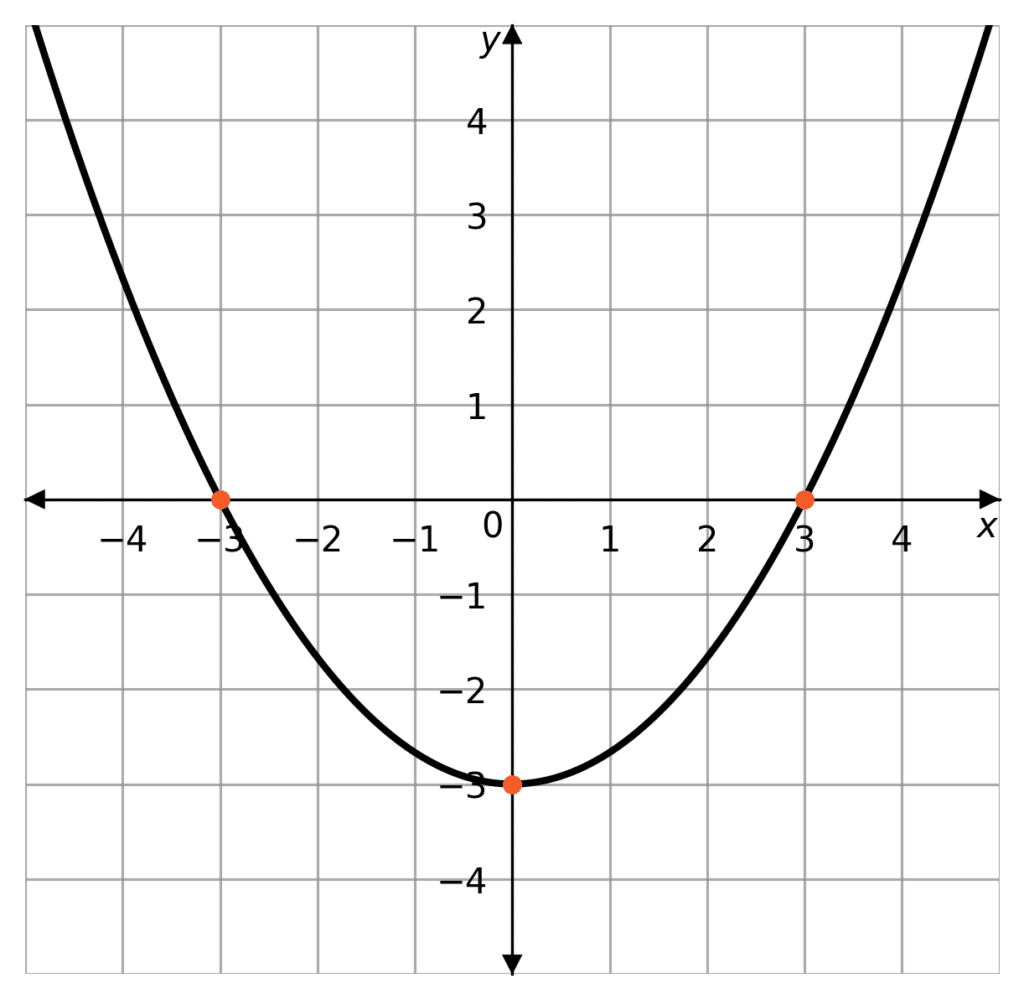In this example, the roots of the quadratic can be interpreted graphically as the points that the curve crosses the $x$-axis. Giving us

$(-3,0)$ and $(3,0)$

So the roots are $x=3$ and $x = -3$

The $y$-intercept is where the curve crosses the $x$-axis. In this case it is at the point

$(0,-3)$

So the $y$-intercept is $y=-3$

The graphical interpretation of the $x$ and $y$-intercepts can also be done for other types of graphs such as cubic graphs.

Level 4-5GCSEAQAEdexcelOCR
Level 4-5GCSEAQAEdexcelOCR

## Reciprocal Graphs

Reciprocal graphs have the general form

$y = \dfrac{\textcolor{red}{k}}{x}$

e.g.,

$y = \dfrac{\textcolor{red}{1}}{x}$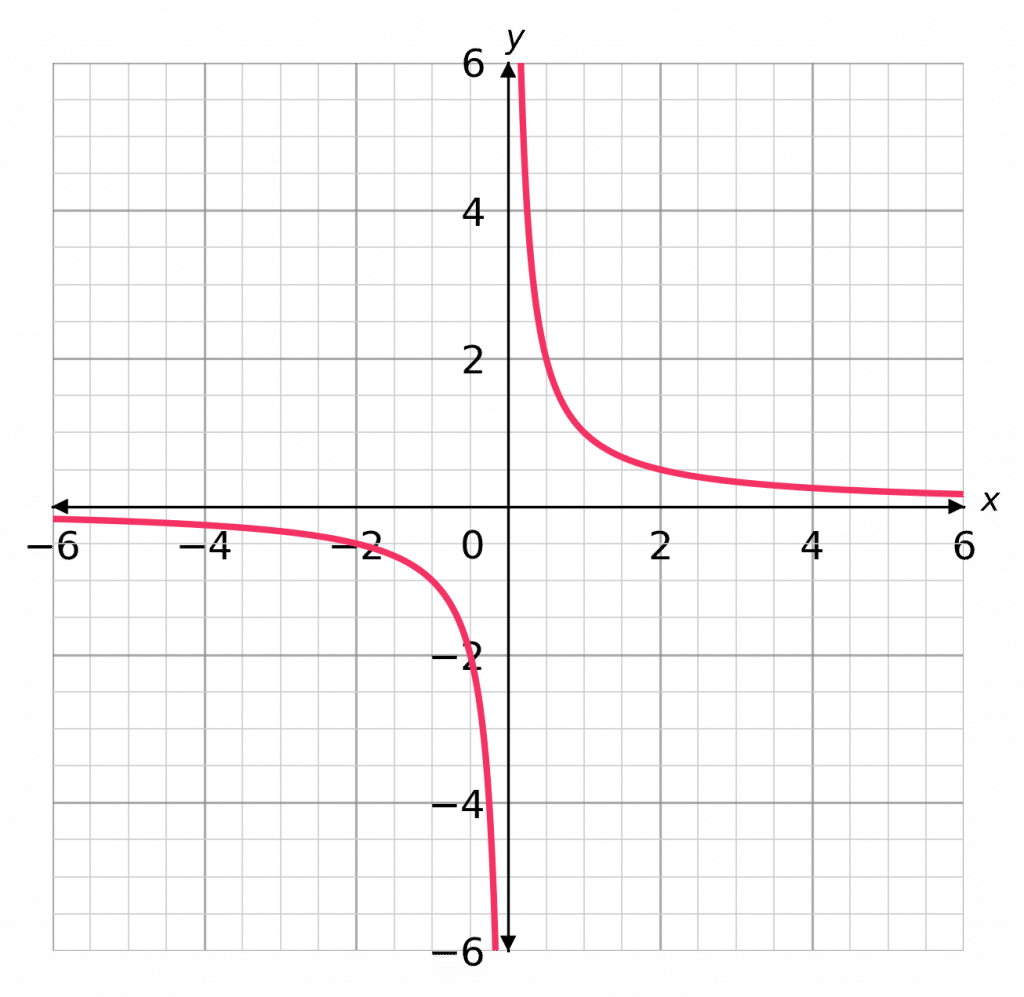Level 4-5GCSEAQAEdexcelOCR
Level 6-7GCSEAQAEdexcelOCR

## Exponential Graphs

Exponential graphs have the general form

$y = \textcolor{blue}{k}^x$

e.g.,

$y = \textcolor{blue}{3}^x$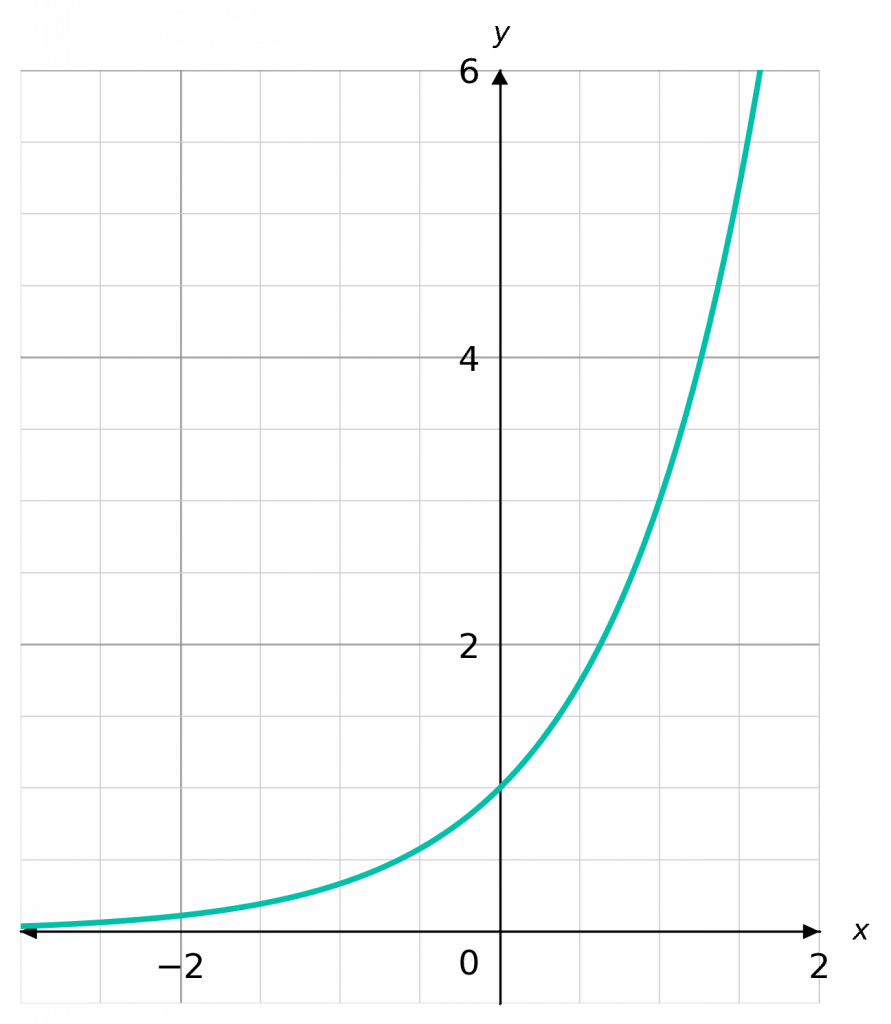Level 6-7GCSEAQAEdexcelOCR
Level 4-5GCSEAQAEdexcelOCR

$y=x^2-x-5$

[2 marks]

First  draw a table of coordinates from $x=-2$ to $x=3$, then use the values to plot the graph between these values of $x$.

Step 1: Draw a table for the values of $x$ between $-2$ and $3$.

Step 2: Substitute our values of $x$ into the equation to get the corresponding $y$ values.

For example, when $x=\textcolor{red}{-2}$, we get

$y=(\textcolor{red}{-2})^2-(\textcolor{red}{-2})-5=4+2-5= \textcolor{blue}{1}$.

Step 3: Continue this process for all other values of $x$Step 4: From the table we get coordinates to plot. e.g. $(\textcolor{red}{-2}, \textcolor{blue}{1})$

Once plotted, we join all the points with a smooth curvegiving the following graph.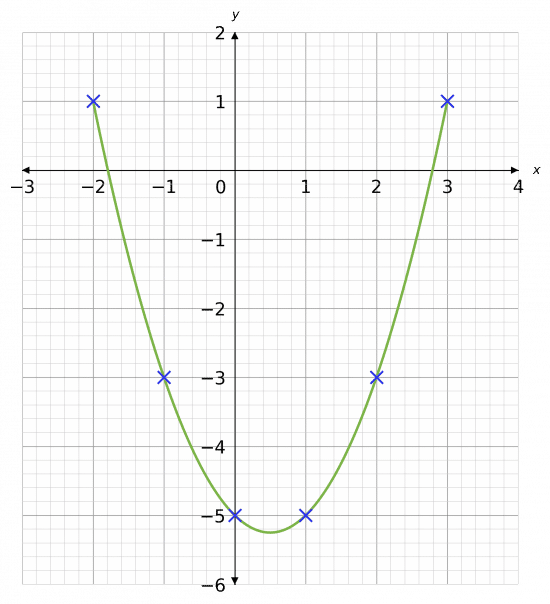Level 4-5GCSEKS3AQAEdexcelOCR
Level 4-5GCSEKS3AQAEdexcelOCR

## Example 2: PlottingCubics

Using the equation $y=x^3-2x^2$, draw a table of coordinates from $x=-1$ to $x=3$. Use the values to plot the graph between these $x$ values.

[3 marks]

Step 1: Draw a table of the coordinates for $x$ from $-1$ to $3$

Step 2: Substitute our values of $x$ into the equation to get the corresponding $y$ values.

For example, for $x=\textcolor{red}{1}$, we get

$y=\textcolor{red}{1}^3-2(\textcolor{red}{1})^2=\textcolor{blue}{-1}$.

Step 3: Continue this process for all other values of $x$Step 4: From the table we get coordinates to plot. e.g. $(\textcolor{red}{1}, \textcolor{blue}{-1})$

Once plotted, we join all the points with a smooth curve giving the following graph.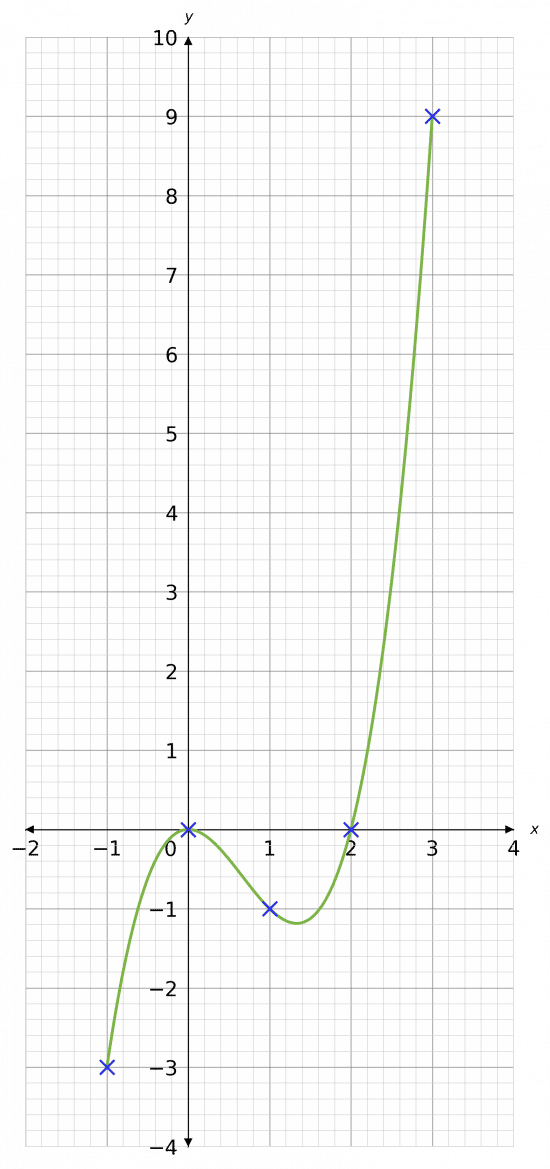Level 4-5GCSEAQAEdexcelOCR

## Example 3: Plotting Reciprocals

Using the equation $y= \dfrac{1}{x}$ draw a table of coordinates from $x = -2.5$ to $x=2.5$. Use the values to plot the graph between these $x$ values.

[3 marks]

Step 1: Draw a table of the coordinates for $x$ from $-2.5$ to $2.5$

Step 2: Substitute our values of $x$ into the equation to get the corresponding $y$ values.

For example, for $x = -2$, we get

$y= \dfrac{1}{-2} =-0.5$

Step 3: Continue this process for all other values of $x$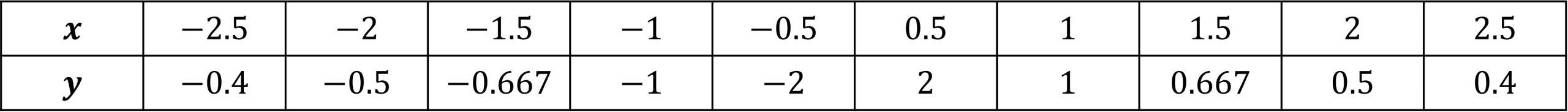Step 4: From the table we get coordinates to plot. e.g. $(-2, -0.5)$

Once plotted, we join all the points with smooth curve giving the following graph.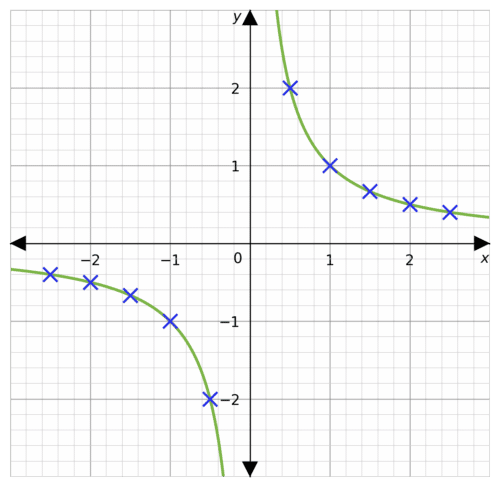Level 4-5GCSEAQAEdexcelOCR

## Example 4: Plotting Exponentials

Using the equation $y= 2^x$ draw a table of coordinates from $x = -1$ to $x=3$. Use the values to plot the graph between these $x$ values.

[3 marks]

Step 1: Draw a table of the coordinates for $x$ from $-1$ to $3$

Step 2: Substitute our values of $x$ into the equation to get the corresponding $y$ values.

For example, for $x = -1$, we get

$y= 2^-1 =0.5$

Step 3: Continue this process for all other values of $x$Step 4: From the table we get coordinates to plot. e.g. $(-1, 0.5)$

Once plotted, we join all the points with smooth curve giving the following graph.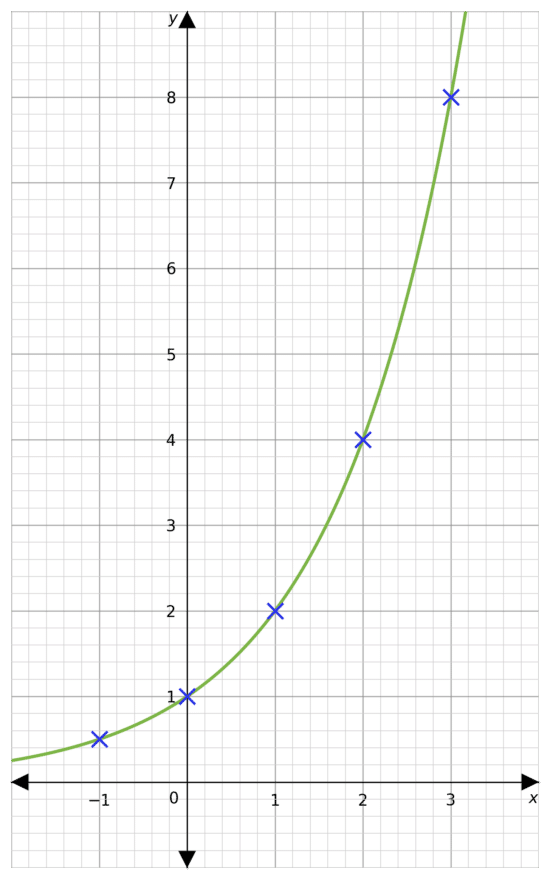Level 6-7GCSEAQAEdexcelOCR

## Quadratics and Harder Graphs Example Questions

We will complete this table by substituting in the values of $x$ to get the missing values of $y$. For example, when $x=2$,

$y=(-4)^2+4(-4)-9=16-16-9=-9$

Continuing this with the rest of the $x$ values, we get the completed table below.Then, plotting these coordinates on a pair of axes and joining them with a curve, we get the graph below.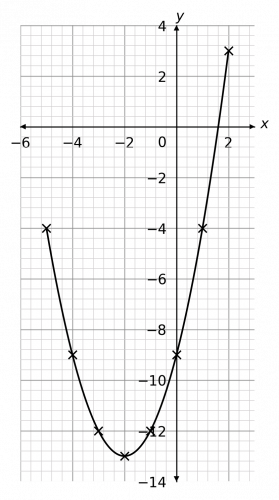Gold Standard Education

We will complete this table by substituting in the values of $x$ to get the missing values of $y$. For example, when $x=-2$,

$y=(-2)^3+3(-2)^2-4=-8+12-4=0$

Continuing this with the rest of the $x$ values, we get the completed table below.Then, plotting these points on a pair of axes and joining them with a curve, we get the graph below.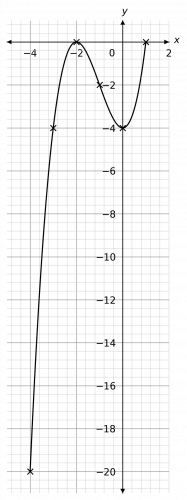Gold Standard Education

We will complete this table by substituting in the values of $x$ to get the missing values of $y$. For example, when $x=2$,

$y=(0.2)^2=0.04$

Continuing this with the rest of the $x$ values, we get the completed table below.Then, plotting these points on a pair of axes (to the best of your ability – some of the $y$ values are so small they’re going to end up practically on the $x$-axis) and joining them with a curve, we get the graph below.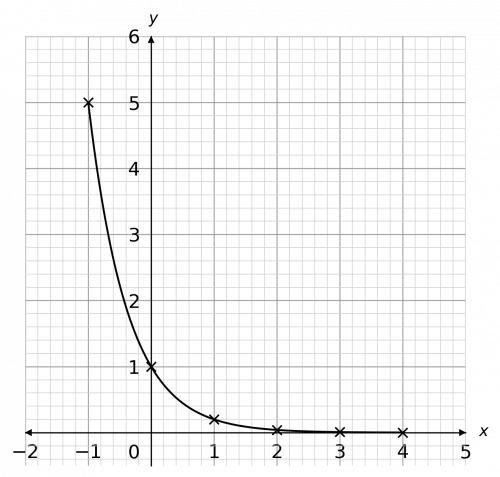Gold Standard Education

We draw this table by substituting the $x$ values into the equation. For example, for $x=1$, we get

$y=2^1=2$.Carrying this on with the rest of the numbers, we get the table above. Then, plotting these points and joining them with a curve, we get the graph to the right.

The exponential graph also has an asymptote along the $x$-axis. Its shape varies very little, except that when the base of the exponential (here, the function is $2^x$ so the base is $2$) is a number between $0$ and $1$, the shape of the graph is a mirror image of this one. Specifically, a reflection in the $y$-axis.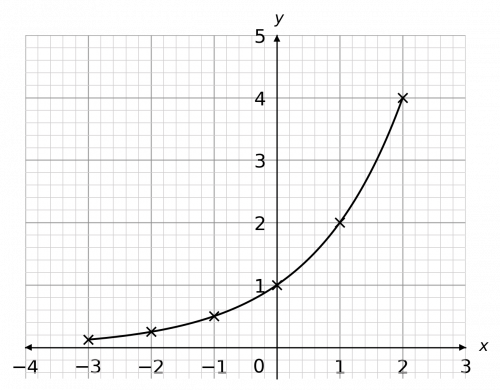Gold Standard Education

We draw this table by substituting the $x$ values into the equation. For example, for $x=2$, we get

$y=\dfrac{1}{2}=0.5$.Then, plotting these points on a pair of axes and joining them with a curve, we get the graph below.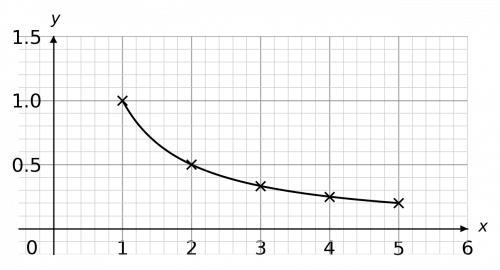Gold Standard Education

## Quadratics and Harder Graphs Worksheet and Example Questions

### (NEW) Plotting Quadratics and Harder Graphs Exam Style Questions

Level 4-5Level 6-7GCSE

## Quadratics and Harder Graphs Drill Questions

Level 4-5GCSE

Level 4-5GCSE

### Plotting Harder graphs

Level 6-7GCSE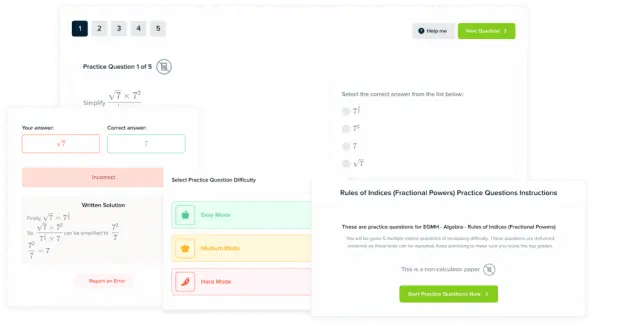Product

### £19.99

/month

Learn an entire GCSE course for maths, English and science on the most comprehensive online learning platform. With revision explainer videos & notes, practice questions, topic tests and full mock exams for each topic on every course, it’s easy to Learn and Revise with the MME Learning Portal.

Level 1-3GCSEKS3

Level 6-7GCSEKS3